TitleCollege Algebra
Answer/Discussion to Practice Problems

Tutorial 5: Rational ExponentsAnswer/Discussion to 1a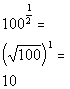*Rewrite exponent 1/2 as a square root

 We are looking for the square root of 100 raised to the 1 power, which is the same as just saying the square root of 100.  If your exponent's numerator is 1, you are basically just looking for the root (the denominator's exponent).  Our answer is 10 since the square root of 100 is 10.Answer/Discussion to 1b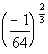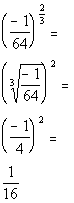*Cube root of -1/64 = -1/4

 In this problem we are looking for the cube root of -1/64 squared.  Again, I think it is easier to do the root part first if possible.  The numbers will be easier to work with.  The cube root of -1/64 is -4 and (-4) squared is 16.Answer/Discussion to 2a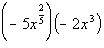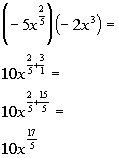Answer/Discussion to 2b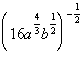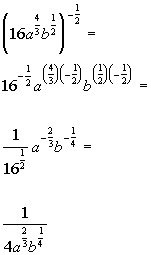*Square root of 16 = 4Answer/Discussion to 2c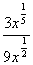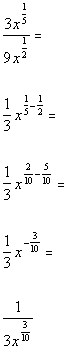Answer/Discussion to 3a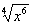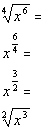*Simplify exponent *Rewrite exponent 3/2 as a square root of x cubed

Last revised on Dec. 6, 2009 by Kim Seward.
All contents copyright (C) 2002 - 2010, WTAMU and Kim Seward. All rights reserved.